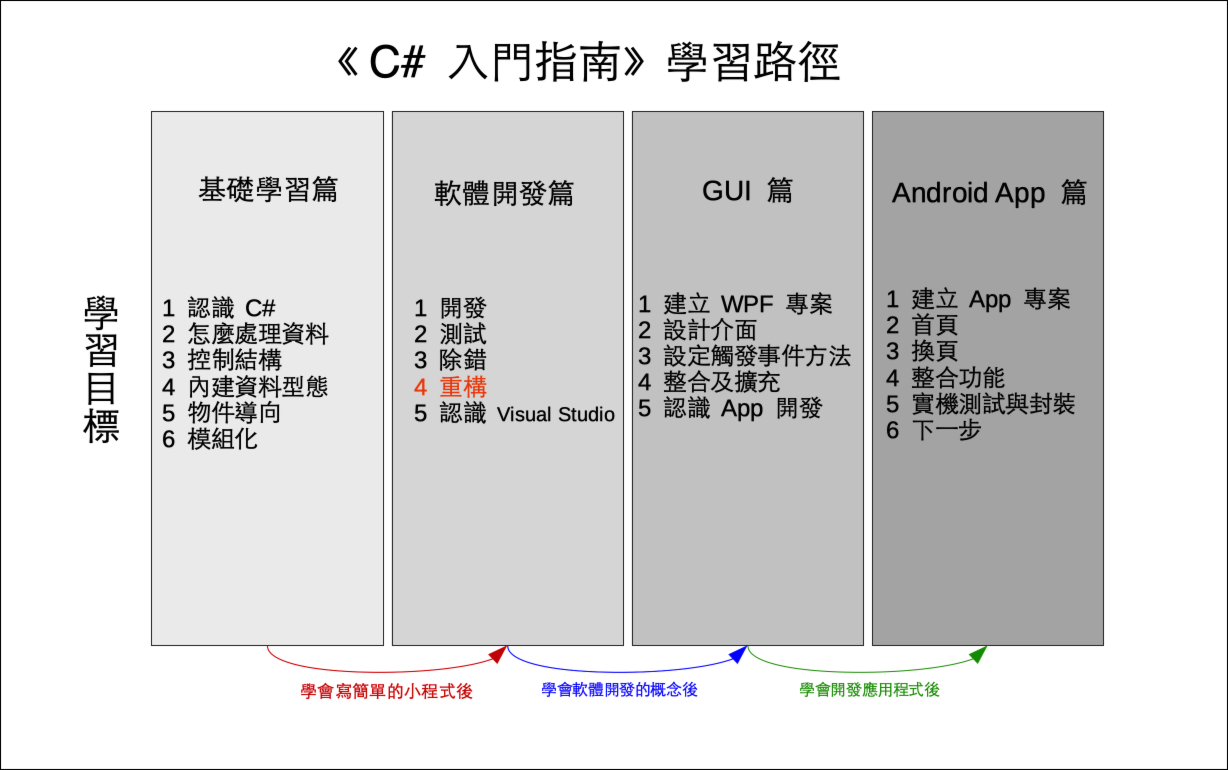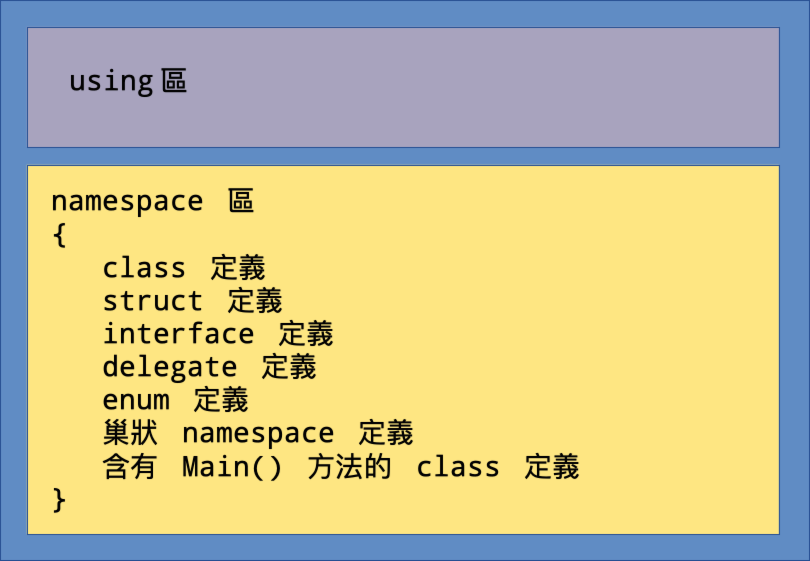# 單元 20 - 程式結構與重構

～～學習進度表～～C# 程式檔案的結構如下圖，主要分成兩大部分using 區」就是引入 .NET 程式庫 (library) 相關內容的地方，「namespace 區」則是程式檔案的相關定義，凡是類別 (class) 、結構 (structure) 、介面 (interface) 等等，都在這裡面定義，如果這個命名空間 (namespace) 需要可以執行，就要定義含有 Main() 方法 (method) 的類別。

1. 開頭引入 .NET 程式庫；
2. 其餘程式相關定義都放在 namespace 裡；
3. 可執行程式要定義含有 Main() 方法的類別。

5 × 10 = 50

1 × 2 × 3 × ⋯⋯ × 25 × 26 = 4.0329 × 1026

``````using System;

namespace EncryptNamespace
{
class Encrypt
{
// 宣告私有欄位
private string _letter, _code;

// 存取 _letter 的屬性
public string Letter {
get {return _letter;}
set {
_letter = value;
}
}

// 存取 _code 的屬性
public string Code {
get {return _code;}
set {
// value 必須是符合英文字母表的字串
if (value is string && value.Length == 26)
{
_code = value;
}
// 不符合條件不做設定，印出錯誤訊息
else
{
Console.WriteLine("密碼字串錯誤，請修改程式。");
}
}
}

// 沒有參數的建構子，建立隨機密碼表
public Encrypt()
{
Letter = "abcdefghijklmnopqrstuvwxyz";
Code = Shuffle(this.Letter);
}

// 有參數的的建構子，由參數建立密碼表
public Encrypt(string newCode)
{
Letter = "abcdefghijklmnopqrstuvwxyz";
Code = newCode;
}

// 攪亂字串順序
string Shuffle(string s)
{
// 將參數字串轉換成字元陣列
char[] s_array = s.ToCharArray();
// 建立隨機物件
Random r = new Random();
// 取得字串長度
int n = s.Length;
// 依序攪亂字元陣列的順序
while (n > 1)
{
n--;
int k = r.Next(n + 1);
var v = s_array[k];
s_array[k] = s_array[n];
s_array[n] = v;
}

// 最後回傳將字元陣列合併的字串
return new string(s_array);
}

// 編碼方法
public string ToEncode(string s)
{
// result 為處理過程中的暫存字串
string result = "";
// 逐一取得字元進行處理
foreach (char i in s)
{
// 判斷是否為英文小寫字母
if (this.Letter.Contains(i.ToString()))
{
result += this.Code[this.Letter.IndexOf(i)];
}
// 不是英文小寫字母，直接將字元附加到 result 之後
else
{
result += i;
}
}
// 回傳結果
return result;
}

// 解碼方法
public string ToDecode(string s)
{
// 建立暫存字串
string result = "";
// 逐一取得字元進行處理
foreach (char i in s)
{
// 判斷是否為英文小寫字母
if (this.Letter.Contains(i.ToString()))
{
result += this.Letter[this.Code.IndexOf(i)];
}
// 不是英文小寫字母，直接將字元附加到 result 之後
else
{
result += i;
}
}
// 回傳結果
return result;
}
}

class Program
{
static void Main(string[] args)
{
// 建立密碼物件 e
Encrypt e = new Encrypt();
// 印出密碼表
Console.WriteLine(e.Code);
// 設定測試字串
string s1 = "There is no spoon.";
// 印出測試字串
Console.WriteLine(s1);
// 進行編碼
string s2 = e.ToEncode(s1);
// 印出編碼後的字串
Console.WriteLine(s2);
// 進行解碼
string s3 = e.ToDecode(s2);
// 印出解碼後的字串
Console.WriteLine(s3);
// 設定長度不符的新密碼物件
Encrypt e2 = new Encrypt("no");
// 設定長度相符的新密碼物件
Encrypt e3 = new Encrypt("qazwsxedcrfvtgbyhnujmikolp");
// 再次進行編碼
string s4 = e3.ToEncode(s1);
// 印出編碼後的字串
Console.WriteLine(s4);
// 再次進行解碼
string s5 = e3.ToDecode(s4);
// 印出解碼後的字串
Console.WriteLine(s5);
}
}
}

//《程式語言教學誌》的範例程式
// http://kaiching.org/
// 專案：EncryptNamespace
// 檔名：Program.cs
// 功能：示範重構後的 Encrypt 類別
// 作者：張凱慶``````

Encrypt 類別中，首先封裝 _letter_code_letter 是英文小寫字母表， _code 則是攪亂順序的密碼表

``````// 宣告私有欄位
private string _letter, _code;``````

``````// 存取 _letter 的屬性
public string Letter {
get {return _letter;}
set {
_letter = value;
}
}

// 存取 _code 的屬性
public string Code {
get {return _code;}
set {
// value 必須是符合英文字母表的字串
if (value is string && value.Length == 26)
{
_code = value;
}
// 不符合條件不做設定，印出錯誤訊息
else
{
Console.WriteLine("密碼字串錯誤，請修改程式。");
}
}
}``````

``````// value 必須是符合英文字母表的字串
if (value is string && value.Length == 26)``````

```// 沒有參數的建構子，建立隨機密碼表
public Encrypt()
{
Letter = "abcdefghijklmnopqrstuvwxyz";
Code = Shuffle(this.Letter);
}

// 有參數的的建構子，由參數建立密碼表
public Encrypt(string newCode)
{
Letter = "abcdefghijklmnopqrstuvwxyz";
Code = newCode;
}```

Shuffle() 方法用來攪亂字串順序

``````// 攪亂字串順序
string Shuffle(string s)
{
// 將參數字串轉換成字元陣列
char[] s_array = s.ToCharArray();
// 建立隨機物件
Random r = new Random();
// 取得字串長度
int n = s.Length;
// 依序攪亂字元陣列的順序
while (n > 1)
{
n--;
int k = r.Next(n + 1);
var v = s_array[k];
s_array[k] = s_array[n];
s_array[n] = v;
}

// 最後回傳將字元陣列合併的字串
return new string(s_array);
}``````

``````// 將參數字串轉換成字元陣列
char[] s_array = s.ToCharArray();``````

``````// 依序攪亂字元陣列的順序
while (n > 1)
{
n--;
int k = r.Next(n + 1);
var v = s_array[k];
s_array[k] = s_array[n];
s_array[n] = v;
}``````

``result += this.Code[this.Letter.IndexOf(i)];``

``result += this.Letter[this.Code.IndexOf(i)];``

``````static void Main(string[] args)
{
// 建立密碼物件 e
Encrypt e = new Encrypt();
// 印出密碼表
Console.WriteLine(e.Code);
// 設定測試字串
string s1 = "There is no spoon.";
// 印出測試字串
Console.WriteLine(s1);
// 進行編碼
string s2 = e.ToEncode(s1);
// 印出編碼後的字串
Console.WriteLine(s2);
// 進行解碼
string s3 = e.ToDecode(s2);
// 印出解碼後的字串
Console.WriteLine(s3);
// 設定長度不符的新密碼物件
Encrypt e2 = new Encrypt("no");
// 設定長度相符的新密碼物件
Encrypt e3 = new Encrypt("qazwsxedcrfvtgbyhnujmikolp");
// 再次進行編碼
string s4 = e3.ToEncode(s1);
// 印出編碼後的字串
Console.WriteLine(s4);
// 再次進行解碼
string s5 = e3.ToDecode(s4);
// 印出解碼後的字串
Console.WriteLine(s5);
}``````

 C:\Encrypt> dotnet run tgkqjpzamvnhedrlbuycwfxois There is no spoon. Tajuj my dr ylrrd. There is no spoon. 密碼字串錯誤，請修改程式。 Tdsns cu gb uybbg. There is no spoon. C:\Encrypt>1. C# 程式設計手冊 | Microsoft Docs - 類別
2. Visual Studio | Microsoft Docs - 重構

1. C# 程式結構主要是定義命名空間，然後在命名空間中放所有的定義，如果這個命名空間是可以執行的，就要在類別中定義 Main() 方法。
2. 重構是指重新整理程式碼，讓程式更易於維護。
3. 常見的重構技術包括整理屬性、方法，挑出類別的共通特性定義父類別等等。

1. 什麼是重構？為什麼要替開發好的程式進行重構？
2. Encrppt 類別進行了哪些重構？為什麼要作這些重構？

1. 承接上一個單元的猜數字遊戲，將新專案寫在 Exercise2001 中，替建構子新增一個參數 digit ，並且將 digit 預設為 4 ，注意呼叫 Substring() 也要改成 digit
2. 承上題，將新專案寫在 Exercise2002 中，將 Guess 類別的欄位 answerab 封裝，並設定相關屬性，注意原本在 Program 類別的 Main() 呼叫的欄位也要同時改成屬性。